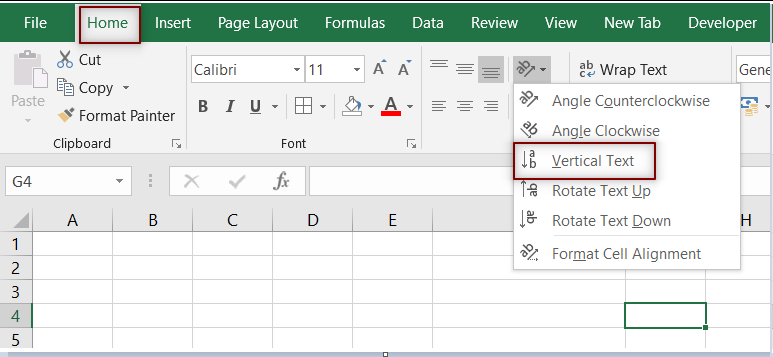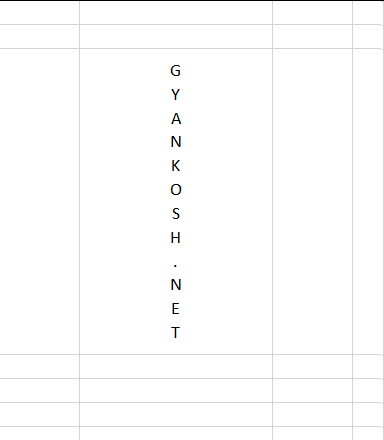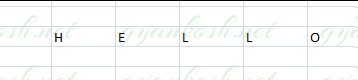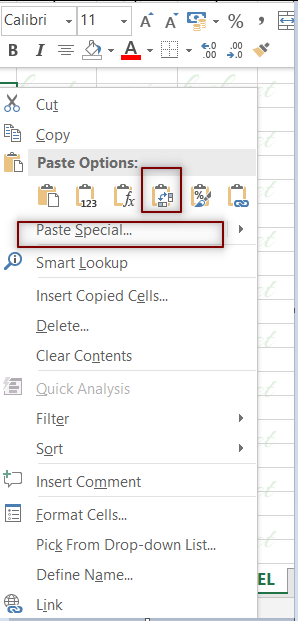PROVIDING ACCURATE, RELIABLE AND STRAIGHT TO THE POINT KNOWLEDGE ABOUT DIFFERENT IT TOOLS EXPLAINED WITH PRACTICAL EXAMPLES

# HOW TO CREATE VERTICAL TEXT IN EXCEL

## INTRODUCTION

Whenever we prepare any report in excel, we have two constituents in any report.

The Text portion and the Numerical portion.

But just storing the text and numbers doesn’t make the super reports. Many times we need to automate the process in the reports to minimize the effort and improve the accuracy.

Many functions are provided by the Excel which work on Text and give us the useful output as well. But few problems are still left on which we need to apply some tricks with the available tools.

THIS WAS AN EXCERPT FROM THE FIRST ARTICLE OF THIS SERIES MANIPULATING TEXT IN EXCEL – PART I

In this articles , we will continue learning many more techniques about the manipulation of text in Excel.

## HOW TEXT IS HANDLED IN EXCEL?

TEXT is simply the group of characters and strings of characters which convey the information about the different data and numbers in Excel. Every character is connected with a code [ANSI].
Text comprises of the individual entity character which is the smallest bit which would be found in Excel.
We can perform the operations on the strings[Text] or the characters.
Characters are not limited to A to Z or a to z but many symbols are also included in this which we would see in the later part of the article.

TEXT IS AN INACTIVE NUMBER TYPE[FORMAT] IN EXCEL. ANYTHING STORED AS TEXT [NUMBER OR DATE] WON’T RESPOND TO ANY STANDARD FORMULAS OR FUNCTIONS BUT SPECIALLY DESIGNED TEXT FUNCTIONS. [EXCEPTIONS DO OCCUR IN CASE OF NUMBERS]
If we need to make anything inactive, such as Date to be non responding to the calculation, we put it as a text. Similarly if we want to avoid any calculations for a number it needs to be put as a text.

## CREATE VERTICAL TEXT IN EXCEL

Let us try to create vertical text in excel.

The vertical text can be the need of any report where we want the heading to be vertical.

TEXT BEING VERTICAL can have two meanings.

1. Vertical text but in the same cell.

2. Vertical text in the different cells.

VERTICAL TEXT IN THE SAME CELL:

Follow the steps to create a vertical text in the same cell.

1. Enter the text normally, where we want to enter.

2. Go to HOME TAB>ORIENTATION>VERTICAL TEXT.3. The text will become vertical simply. Check out the following picture for the results.2.VERTICAL TEXT IN DIFFERENT CELLS:

If the text is in cells horizontally and we want to place it vertically we can use the following methods.

Let us take an example where the following text is put in the cells horizontally.

HELLO as shown in the picture below.

USING PASTE SPECIAL

USING TRANSPOSE FUNCTION.

USING PASTE SPECIAL:STEPS TO MAKE THE TEXT VERTICAL USING THE PASTE SPECIAL FUNCTION

• Select all the cells containing the letters or words.
• Right Click the cell where we want to put the text vertically.
• Choose transpose option directly or paste special.• The result will appear.
• Instead if we go for PASTE SPECIAL. The paste special dialog box will open.
• Choose TRANSPOSE and click OK.

Click OK.

The result will appear as the word HELLO will become vertical .

### USING TRANSPOSE FUNCTION.

GIVEN TEXT- HELLO as shown in the picture below.STEPS TO MAKE THE TEXT VERTICAL USING THE TRANSPOSE FUNCTION.

• Select the exact number of cells in the same layout of the actual data which needs to be transposed i.e. with the same number of rows to be converted to same number of columns at a new place. Look at the picture above. We selected the exact 5 vertical cells before applying the function.(D18 TO H18 in this case)
• Now type the formula =TRANSPOSE(D18:H18) but don’t PRESS ENTER.
• Press CTRL+SHIFT+ENTER.
• The text will become vertical.

• ## WHAT IS EXCEL, HOW EXCEL WORKS, WRITE FORMULA IN EXCEL, EXAMPLE OF USING FORMULA IN EXCEL, ANIMATED EXAMLPE OF USING FORMULA IN EXCEL

LEARN

• EXCEL
• JAVASCRIPT
• MORE TO COME…

Encourage us by following at …

If you have any queries or suggestions or any problem which you would like us to solve for you, kindly mail at

[email protected]